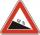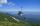# Unit conversion + arctangent - math problems

#### Number of problems found: 5Road sign informs the gradient is 10.9%. Calculate the angle which average decreases.Between cities A and B is route 13 km long of stúpanie average 7‰. Calculate the height difference of cities A and B.
• Slope of trackCalculate the average slope (in permille and even in degrees) of the rail tracks between Prievidza (309 m AMSL) and Nitrianske Pravno (354 m AMSL), if the track is 11 km long.
• Map - climbOn the map of High Tatras in scale 1:11000 are cable car stations in the Tatranska Lomnica and in the Skalnate Pleso with distance 354.6 mm. Altitude of this stations are 949 m and 1760 m. What is average angle of climb of this cable car track?
• Sphere in coneA sphere is inscribed in the cone (the intersection of their boundaries consists of a circle and one point). The ratio of the surface of the ball and the contents of the base is 4: 3. A plane passing through the axis of a cone cuts the cone in an isoscele

We apologize, but in this category are not a lot of examples.
Do you have an interesting mathematical word problem that you can't solve it? Submit a math problem, and we can try to solve it.

We will send a solution to your e-mail address. Solved examples are also published here. Please enter the e-mail correctly and check whether you don't have a full mailbox.

Please do not submit problems from current active competitions such as Mathematical Olympiad, correspondence seminars etc...

Unit conversion - math word problems. Arctangent - math word problems.